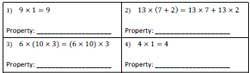# Multiplication Properties Worksheets

The printable multiplication properties worksheets in this page contain commutative and associative property of multiplication; distributive property; identifying equivalent statement; multiplicative inverse and identity; and more. The pdf worksheets cater to the learning requirements of children in grade 3 through grade 6. Some of these worksheets are absolutely free of cost.

## Basic Multiplication Properties

Commutative Property of Multiplication

Identify the correct choice from the list of multiplication properties.Commutative Property 2

Associative Property of Multiplication

Associative Property: a * (b * c) = (a * b) * c

Find out which of the following choices describes associative property of multiplication.

Associative Property 2

## Distributive Property Worksheets

Identify the Equivalent Statement

You have 4 choices with 3 distractors in each question. Identify the correct equivalent statement.

Equivalent Statement 2

Distributing Numbers

Apply distributive principle of multiplication to rewrite the multiplication sentence.

Distributive Property Worksheet 2

Solve using Distributive Property

Use distributive property to solve the multiplication problems in these printable worksheets for 3rd grade and 4th grade kids.Solving Worksheet 2

## Multiplication Properties: Special Focus

Missing Number

Use commutative and associative property of multiplication to find the missing number in these grade 4 and grade 5 exercises.

Missing Number 2

Use multiplicative identity and multiplicative inverse to find the missing number.

Identity or Inverse: Moderate

Multiplicative Inverse

Multiplicative inverse of a is 1/a; a/b is b/a and vice versa. 5th grade and 6th grade children need to identify the multiplication inverse for each problem.

Multiplicative Inverse: Moderate

Multiplicative Inverse: Difficult

Identifying the Property

Multiplication properties worksheet pdfs include multiplication equation in each question. Identify what property is used in each question.Multiplication Property Worksheet 2

Properties Worksheets: Mixed Review

It includes both addition and multiplication properties in each worksheet.

Properties Worksheets

Related Worksheets